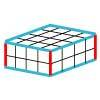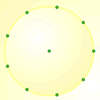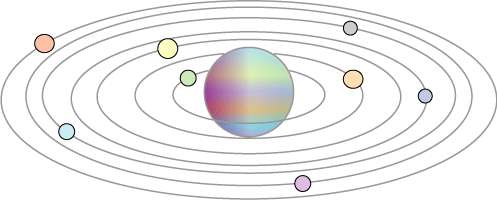#### You may also like### Round and Round the Circle

What happens if you join every second point on this circle? How about every third point? Try with different steps and see if you can predict what will happen.### Making Cuboids

Let's say you can only use two different lengths - 2 units and 4 units. Using just these 2 lengths as the edges how many different cuboids can you make?How many DIFFERENT quadrilaterals can be made by joining the dots on the 8-point circle?

# The Moons of Vuvv

##### Age 7 to 11Challenge Level

The planet of Vuvv has $7$ moons which lie spread out on one plane in a great disc round it. These Vuvvian moons all have long and confusing names so scientists usually call them by their initials: $A, B, C, D, E, F$ and $G$ starting from the nearest one to the planet.When two of these moons line up with the planet it is called a 'lunar eclipse'. When three line up with the planet it is called a 'double eclipse', when four do it is a 'triple eclipse' and so on. Once in a while all seven moons line up with the planet and this is called a 'super-eclipse'.

Moon $A$ completes a cycle round the planet in one Vuvvian year, moon $B$ takes two years, moon $C$ takes three years, moon $D$ takes four years and so on.

How long is it between each 'super-eclipse' on the planet of Vuvv?